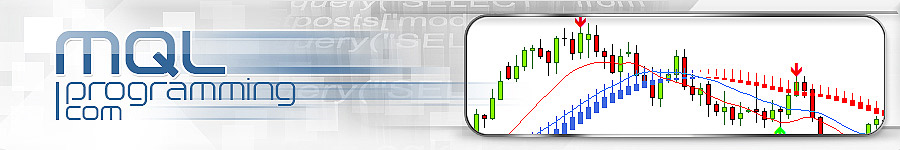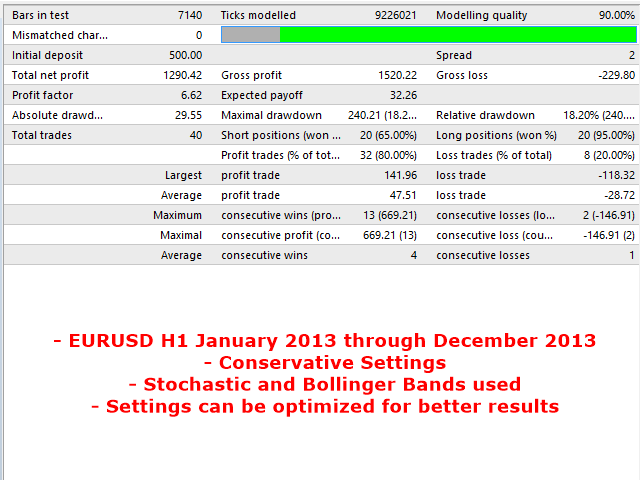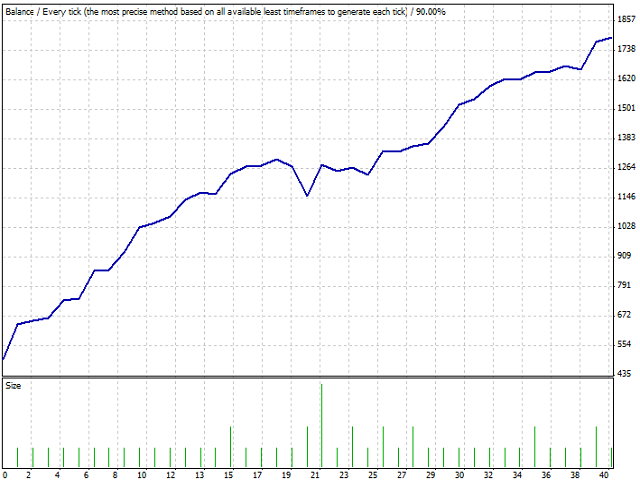| SHARE | REQUEST A QUOTE | FREE | PRODUCTS | HOME# MQL Universal EA

SummaryThis EA will trade using standard MT4 indicators such as Moving Averages, MACD, RSI, Bollinger Bands, and so on. The EA was made so that these indicators can be mixed or matched so a number of possibilities can be traded with. Each indicator can be enabled or disabled and many settings are adjustable for each indicator. There are also other features included like multiple time frame capability and money management. As a result, many different trading strategies can be traded from this single EA. Trading results will depend on the strategy and settings you choose to use.

Inputs
• Stealth Mode
If true, all TP/SL levels will be hidden from the broker and will not be visible in the trading terminal. If false, orders will be modified and the TP/SL levels will be entered with the broker as usual.
• Close By Opposite
If true, an opposite trading signal will automatically close out an order if it is still open. For example, if a buy order is open and a new sell signal is recognized, then the buy trade would automatically close.
• TakeProfit
Amount in pips that the TP will be set at for each trade.
• StopLoss
Amount in pips that the SL will be set at for each trade.
• Slippage
The maximum amount of slippage allowed for opening and closing a trade. If the slippage at the time of execution is higher than this amount then the execution will stop.
The maximum amount of spread allowed for opening and closing a trade. If the spread is higher than this amount, the order will not be executed. The EA will wait until the spread drops down to allowable levels and try again as long as all indicator conditions are still met.
• MagicNumber
This is a typical Magic Number input for the EA. Set a different number of your choice for each chart you have an EA running on. The magic number must be greater or equal to 0 and must be a whole number.
• Lot Mode
This is where you should select the type of money management (MM) that you want to use. The available options are : 1=Fixed, 2=Risk, 3=Martingale.
• Fixed Lot Size
This input is used if the 'Lot Mode' is set to 1.
• Risk
This input is used if the 'Lot Mode' is set to 2. The higher the number then the higher the lots per trade. This type of MM uses account equity and account free margin in its calculations.
• Max. Martingale Orders
This input is used if the 'Lot Mode' is set to 3. This input represents the maximum number of times the Martingale multiplier will be applied to the lot size.
• Martingale Multiplier
This input is used if the 'Lot Mode' is set to 3. The input represents the multiplier used for the Martingale trades. For example, if the last losing trade's lot size was 2.00 and the multiplier is set to 2 then the new trade's lot size would be 4.00.
• Trailing Start
The amount of profit in pips that must be reached before the TS is activated. For example, if set to 10 then this means that once 10 pips in profit is reached, the trailing stop will be started and the SL will be moved 'Trailing Stop' pips behind current market price. Setting this input to 0 does not disable the TS. Setting to 0 would simply mean that the TS is immediately started once a pip in profit is reached.
• Trailing Stop
The amount of pips that the SL will trail the market. If set to 0, then the trailing stop will be disabled.
• Trailing Step
The amount of pips difference between each TS modification. For example, if set to 1 then the SL might be modified like this: 1.5001, 1.5002, 1.5003, and so on.
• Break Even Start
The amount of pips in profit an order must go before the BE is activated. The BE is disabled if this input is 0.
• Break Even Pips
The amount of the break even relative to the order's open price. For example, if a buy order opens at 1.5000 and this input is set to 1, then the BE would move the SL to 1.5001 so that 1 pip profit would be locked in.
• Use Timer
If true, the trade timer is enabled. If false, the trade timer is disabled.
• Timer
This represents the allowable time period that new trades can be opened. Existing trades will not be closed out if the current time goes outside the timer limits. They would still closeout normally. Only new trades will not be made outside the allowed times. This input must be in a valid format in order to be used properly. The format should be similar to the default setting. Do not add any strange characters or spaces to the input string. For example, 08.00 and 08 00 are not the same as 08:00. The timer is based on the broker's operating time as seen in the MetaTrader platform. The timer is not based on GMT time or your PC's time. Both hours and minutes can be used in the timer. This input is only used if the 'UseTimer' input is true.
• Indicators Shift
This input is used for all enabled indicators. It represents the shift that the EA will look at the indicator values. If set to 0, then the EA will consider real-time values. If set to 1, then the EA will look at the last closed bar's values. And so on. Setting this input greater than 0 will make the EA use confirmed indicator values.
• Reverse Signals
This input is used for all enabled indicators. It will reverse the signal recognized for each indicator. For example, if the indicator normally is showing a buy signal and this input is true, then the EA would see it as a sell signal instead.
• MA's Used
Turns on/off the MA signal. The MA signal is when the faster MA is crossed over the slower MA. For example, if the faster MA is higher than the slower MA then this is a buy signal.
• MACD Used
Turns on/off the MACD signal. The MACD signal is when the main MACD histogram bars are crossed over the MACD signal line. For example, if the main histogram bars are above the signal line then it is a buy signal.
• STOCH Used
Turns on/off the Stochastic signal. The Stochastic signal is when the main line is crossed over the signal line. For example, if the main line is above the signal line then this is a buy signal.
• RSI Used
Turns on/off the RSI signal. The RSI signal is when the RSI value is crossed above or below the 'RSI_High_Level' and the 'RSI_Low_Level'. For example, let's say the 'RSI_Low_Level' is set to 30 and the 'RSI_High_Level' is set to 70. And the RSI is 31.55 and the last cross was a cross upwards of the low line. Then this is a buy signal since the RSI is higher than the low level but lower than the high level and heading upwards.
• CCI Used
Turns on/off the CCI signal. The CCI signal is when the CCI value is above or below the 'CCI_High_Level' and the 'CCI_Low_Level'. For example, let's say the 'CCI_Low_Level' is set to -100 and the 'CCI_High_Level' is set to 100 . And the CCI is -65.92 and and the last cross was a cross upwards of the low line. Then it would be a buy signal since the CCI is greater than the low level but lower than the high level and heading upwards.
• WPR Used
Turns on/off the WPR signal. The WPR signal is when the WPR value is above or below the 'WPR_High_Level' and the 'WPR_Low_Level'. For example, let's say the 'WPR_Low_Level' is set to -80 and the 'WPR_High_Level' is set to -20. And the WPR is -77.52 and the and the last cross was a cross upwards of the low line. Then it would be a buy signal since the WPR is greater than the low level but lower than the high level and heading upwards.
• BB Used
Turns on/off the Bollinger Bands signal. The Bollinger Bands signal is when price moves higher or lower than the upper and lower Bollinger Bands. For example, if price goes below the lower Bollinger Band then this is a buy signal.
• ENV Used
Turns on/off the Envelopes signal. The Envelopes signal is when price moves higher or lower than the upper and lower Envelope bands. For example, if price goes below the lower Envelope band then this is a buy signal.
• AL Used
Turns on/off the Alligator signal. For a buy signal, the Alligator signal is when the Lips line is higher than the Teeth line while the Teeth line is higher than the Jaws line. And the opposite for a sell signal.
• OSMA Used
Turns on/off the OSMA signal. The OSMA signal is when the OSMA value is crossed above or below the 0 line. For example, if the OSMA value is 0.0014 then this is a buy signal. If the value is -0.0014 then this is a sell signal.
• AO Used
Turns on/off the Awesome Oscillator signal. An Awesome Oscillator signal is when there is a "saucer" formation. A "saucer" consists of two increasing bar values that are above 0 having a bar with lesser value between them. Therefore, an "inverted saucer" consists of two decreasing bar values below 0 having a bar with larger value between them. A "saucer" formation requires a minimum of 3 closed candles.

Notes
• DLL imports must be enabled for this EA to work.
• Each indicator has it's own time frame setting. So the EA has MTF capability.
• Each indicator has it's own applied prices setting. For example, SMA, EMA, SMMA, and LWMA.
• Each indicator has it's own calculation method setting. For example, Close, Open, High, Low, Median, Typical, and Weighted.
• The indicators have a choice of how they will be used:
• The first is 'Confirmation'. In this mode, the indicator will act as confirmation for the order. For example, let's say the Stochastic is enabled and it is set to 'Confirmation'. Then if the main line is already above the signal line then a buy trade can occur. Or let's say the RSI is enabled and set to confirmation. Then if the RSI is already above the low level then a buy trade can occur.
• The second choice is 'Trigger'. If the input is set to 'Trigger' then this means that there needs to be a fresh signal for a trade. For example, let's say Stochastic is enabled, if the main line crossed over the signal line now then a trade will occur (granted other signals and conditions are met of course).

Forum

• The official forum to post your comments, questions, concerns, or bug reports about the MQL Universal EA can be found at Forex Zone Forum.

Example